tech stuff  |

# Wireless Calculators

These calculators are written in Javascript (saves our bandwidth). Find the calculation you want, and enter the various parameters required then click the 'Calculate' button. If you don't like 'em or think you can improve 'em, the code for the calculators is here. For the equations used in each calculator follow the Calculations and equations used link.

Groveling apology 1: The Fresnel calculator said it would default to the mid-point if no obstacle distance was specified and it didn't. More importantly the 60% rule-of-thumb distance was incorrect on the pessimistic side. Thanks to Phil Wells for pointing out the errors.

Groveling apology 2: The Fresnel calculator provides the earth's height at the mid-point. However the ATIS Committe T1A1 recommends use of the effective earth radius (4/3 * earth radius). Previous calculations used the earth's radius and were higher by ~30%. Thanks to Ranjan Sonalkar for pointing out the error.

Partial Groveling apology 3: The Fresnel calculator incorrectly computed the 60% obstacle free zone for MSIE browsers. Those of you using Gecko/Moz based browsers (for example Firefox) had the right answer all the time. And the moral of this tale - get a decent browser. Thanks to Attila Altay Yavuz for pointing out the error. Also the Fresnel calculator equations quoted 72.1 as the conversion factor for miles - this should have been 72.6. Since the calculator normalizes to km/meters this had no effect on results. Thanks to Chris Herrington for pointing out this error. The calculators verify the various values entered for being within a valid range and output an error message if this is not the case. A number of error messages were inconsistent with the ranges being tested. While confusing, these inconsistent messages had no effect on the acuracy of results. Our thanks to Jay Joffe for taking the time to point out this problem.

### A Wireless System (more or less!)Key: Green is good, Red is bad.

1. Power Budget - total power of your system in dBm.
2. Free Space Loss - power loss over distance.
3. System Performance - How far will it go, will it get there or what is my Operating Margin?
4. Fresnel Zones - How much more than LOS or how high should my antenna be?
5. Power Conversion - dBm to milliWatts and milliWatts to dBm

Serious Health Warning: It is nothing short of a minor miracle of physics that ANY wireless signal gets to its destination. These calculators use standard algorithms which give reasonable results BUT it should be borne in mind you can have two locations 1 meter apart in which one gets great reception ALL the time and the other NEVER gets reception. Use this link for our quick overview of the background to radio propagation and the side-bar links for some 'real brain-hurting stuff'. With suitable apologies to the memory of the late, lamented, Douglas Noel Adams the answer to the question of 'how far will this wireless transmission go?' is 42.

Power Budget

Use this calculator to walk through all the factors that make up your Power Budget. The 'Power Budget' is the the total power being output from the wireless system and is the sum of:

• PLUS Radio Transmit Power (A above)
• MINUS cables and connectors losses (B above)
• PLUS antenna gain (C above).
Enter one parameter in each column (or leave blank) depending on what you know and the calculator will supply the appropriate conversions automatically. Up to 2 decimal places may be entered as 0.xx or .xx.

Note: Connector loss is generally small UNLESS you've got lots of 'em in which case you are probably in trouble anyway, or your cable is VERY short. Just leave the line blank if you are feeling lazy.

Calculations and equations used.
 Radio and Antenna Transmit Power Antenna Gain Power mWOR  dBm dB(i) dB Cable Loss Cable Properties (per 100 ft or m) Cable Length dB (100 ft) OR  dB (100 m) feet OR  meters dB Connector Loss Frequency in MHz No. of Connectors MHz. No. dB Budget dB

Free Space Loss

Loss of power over distance (assuming no FRESNEL Effect and nothing in the way). This a very idealised calculation and in practice everything interferes with the signal but it will give you a reasonable approximation of the actual loss over distance.

Enter the system Frequency in MHz and the distance in either Kilometers (Km) or Miles below and then click the 'Calculate' button. 1 GHz = 1000 MHz e.g. 2400 = 2.4 GHz.

Calculations and equations used.
 Frequency Distance Result MHz KmOR  Miles dB

Fresnel Zones

Defines how much clearance you need (yes you need more than simple Line-of-Sight) and for longer links > 3 Km (2 miles) whether you may have a ground clearance problem from our friendly planet. M. Fresnel and his theories.

Enter the Total link distance (in Miles or Kilometers), if you do not enter an Obstacle distance (in Miles or Kilometers) the calculator will use the mid-point for all calculations (Note: assumes antennas at same height). Finally enter the system Frequency in MHz and then click the 'Calculate' button. 1 GHz = 1000 MHz e.g. 2400 = 2.4 GHz.

The calculator will generate the radius of the 1st Fresnel zone only (at the obstable point or the mid-point), the 60% (no obstacle) radius and the height of the effective earth curvature at the mid-point of the Total link distance using the effective earth radius.

Calculations and equations used.
 Total Link Obstacle Distance 1st Fresnel Zone Radius KmOR  Miles KmOR  Miles mat  Km ftat  Miles Frequency 60% No Obstacle Radius MHz m ft Earth Height (mid-point) m ft

System Performance

This calculator will give you one of three answers:

1. If you leave distance blank it will apply the defined SAD factor (or default to 30%) to the Operating Margin and supply the max. distance (in Km and Miles) at which the Margin operates.
2. If you enter the distance it will calculate the Operating Margin and the SAD factor.
3. If you enter distance but leave RX or TX antenna gain (or both) blank it will apply the chosen SAD factor (or default it to 30% if none supplied) and generate the required antenna power. If both are left blank it will calculate a symmetrical antenna gain.
To RESET any parameter above just set to BLANK before clicking Calculate

Notes: RX Sensitivity is ALWAYS expressed as a negative dBm (- dBm) and is the lowest power of signal your radio can handle. Its buried somewhere in your radio spec and will be typically in the range of -80 to -110 dBm. Don't guess or 'fudge' this number.

Note: If you solve for TX antenna (you leave it blank) and define a specific SAD factor it is applied to the TX budget and any TX antenna value will include this factor. If you then click Calculate again without changing anything. the SAD factor will be calculated to include the supplied TX antenna value and thus may show a lower value than the original one.

Calculations and equations used.
 Frequency Distance Result MHz KmOR  Miles (FS Loss) dB TX Power TX Cable TX Antenna dBmOR  mW dBm dB dB RX Sensitivity RX Cable RX Antenna dBm dB dB dB Margin RX Power SAD Factor Theoretical Margin dBm % dB

milliWatts to dBm (and vice versa)

Power in milliWatts to dBm (and vice versa) . Enter the 'Transmit Power' (A or G above) in milliWatts OR the 'Power Ratio' in dBm and click the appropriate 'Calculate' button. 1 Watt = 1000 milliWatts.

Calculations and equations used.
 Transmit Power Result mW dBm Power Ratio Result dBm mW

# Power Budget Calculations

1. mW to dBm = 10Log10(mW)

2. feet to meters = .3048

3. meters to feet = 3.28

4. Calculator normalises all distances to kilometers and meters

5. Connectors loss = 0.10 * square root (frequency in GHz)

# Free Space Calculations

1. Free space loss = 36.56 + 20Log10(Frequency) + 20Log10(Distance in miles)

2. Calculator normalises all distances to miles

3. Miles to Kilometers = 1.609

4. Kilometers to miles = 0.621

# Fresnel Zone Calculations

1. Calculator normalises all distances to kilometers and meters

2. Miles to Kilometers = 1.609

3. Kilometers to miles = 0.621

4. feet to meters = .3048

5. meters to feet = 3.28

6. 1st Fresnel Zone radius (Km) = 17.3 x Sqr root ((Obstacle Distance x (Total Link - Obstacle Distance)) / (Frequency in GHz x Total Link))

7. 1st Fresnel Zone radius (miles) = 72.6 x Sqr root ((Obstacle Distance x (Total Link - Obstacle Distance)) / (Frequency in GHz x Total Link))

9. Radius of nth Fresnel zone (meter) = sqr root ( (n x wave length x Obstacle distance x (Total Link - Obstacle Distance)) / Total Link )

10. wave length (meters) = speed of light (299,792,458 m/s) / frequency in Hz

11. Earth curvature calculation = (Total Link) 2 /(8 * effective earth radius)

13. Earth radius = 3963 miles, 6378 Km

# System Performance Calculations

1. Calculator normalises all distances to miles and feet during calculations.

2. Free space loss = 36.56 + 20Log10(Frequency) + 20Log10(Dist in miles)

3. mW to dBm = 10Log10(mW)

4. dBm to mW = 10(dBm/10)

5. RX Power = Margin - RX sensitivity

6. Theoretical margin = TX power budget + RX power budget - free space loss

7. SAD factor = Theoretical margin/TX power budget * 100 and shows the percentage of spare power on transmission.

# dBm to milliWatts Calculations

1. mW to dBm = 10Log10(mW)

2. dBm to mW = 10(dBm/10)

Note: We had previously titled this section dBm to Watts Calculations which was somewhat confusing since the calculators all use dBm to mW or mW to dBm values. We then compounded the confusion by mixing the equations for mW and Watts. In all cases the calculators did not confuse the two and were correct. For the insatiably curious everywhere, or those with a unique need, the Watts based equations (which are not used in the calculators) are: Watt to dBm = (10Log10(Watts)) + 30; dBm to Watts = 10((dBm - 30)/10).

Problems, comments, suggestions, corrections (including broken links) or something to add? Please take the time from a busy life to 'mail us' (at top of screen), the webmaster (below) or info-support at zytrax. You will have a warm inner glow for the rest of the day.

Tech Stuff

If you are happy it's OK - but your browser is giving a less than optimal experience on our site. You could, at no charge, upgrade to a W3C standards compliant browser such as Firefox

Search

 web zytrax.com

Share

Icons made by Icomoon from www.flaticon.com is licensed by CC 3.0 BY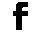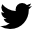Page

Wireless

Theory Stuff

Interesting Stuff

Bibliographies

Standards

Frequencies

Our Pages

Site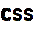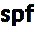Copyright © 1994 - 2021 ZyTrax, Inc. All rights reserved. Legal and Privacy site by zytrax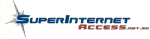web-master at zytrax Page modified: October 21 2015.